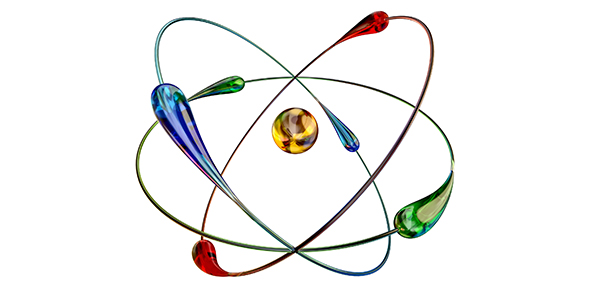# Chapter 33: Atomic Nucleus And Radioactivity

68 Questions | Total Attempts: 187Settings• 1.
X-rays may be regarded as
• A.

High frequency sound waves.

• B.

• C.

Both of these

• D.

None of these

• 2.
X-rays are similar to
• A.

Alpha rays.

• B.

Beta rays.

• C.

Gamma rays.

• D.

All of these

• E.

None of these

• 3.
Which radiation has no electric charge associated with it?
• A.

Alpha rays

• B.

Beta rays

• C.

Gamma rays

• D.

All of these

• E.

None of these

• 4.
Both X-rays and gamma rays are emitted from
• A.

Different parts of the atom.

• B.

A variety of certain atomic nuclei.

• 5.
The sources of X-rays and gamma rays, respectively, are
• A.

Electron clouds and the atomic nucleus.

• B.

The atomic nucleus and electron clouds.

• C.

Both electron clouds.

• D.

Both the atomic nucleus.

• E.

None of these

• 6.
A nucleon is either
• A.

A positron or an electron.

• B.

A proton or an electron.

• C.

A neutron or an electron.

• D.

A proton or a neutron.

• 7.
The mass of an atomic nucleon is nearly
• A.

Twice the mass of an electron.

• B.

Four times the mass of an electron.

• C.

A thousand times the mass of an electron.

• D.

Two thousand times the mass of an electron.

• 8.
Once an alpha particle is outside the nucleus it is
• A.

Free to wander about the nucleus.

• B.

Quickly bound to a neighboring nucleus.

• C.

Electrostatically repelled.

• D.

• 9.
When a nucleus emits a beta particle, its atomic number
• A.

Remains constant, but its mass number changes.

• B.

Remains constant, and so does its mass number.

• C.

Changes, but its mass number remains constant.

• D.

Changes, and so does its mass number.

• E.

None of these

• 10.
A quark is
• A.

An elementary particle.

• B.

A building block of nucleons.

• C.

• D.

All of these

• E.

None of these

• 11.
An atom with an imbalance of electrons to protons is
• A.

• B.

A baryon.

• C.

An ion.

• D.

An isotope.

• E.

None of these

• 12.
The atomic number of an element is the same as the number of its
• A.

Protons.

• B.

Neutrons.

• C.

Nucleons.

• D.

Neither of these

• 13.
The atomic mass number of an element is the same as the number of its
• A.

Protons.

• B.

Neutrons.

• C.

Nucleons.

• D.

None of these

• 14.
Deuterium and tritium are both
• A.

Forms of hydrogen.

• B.

Isotopes of the same element.

• C.

Both of these

• D.

Neither of these

• 15.
Different isotopes of an element have different numbers of
• A.

Protons.

• B.

• C.

Photons.

• D.

Neutrons.

• E.

None of these

• 16.
Electric forces within an atomic nucleus tend to
• A.

Hold it together.

• B.

Push it apart.

• C.

Neither of these

• 17.
Generally speaking, the larger a nucleus is, the greater its
• A.

Stability.

• B.

Instability.

• C.

Neither stability nor instability

• 18.
The half-life of an isotope is one day. At the end of two days the amount that remains is
• A.

None.

• B.

One-half.

• C.

One-quarter.

• D.

One-eighth.

• E.

None of these

• 19.
The half-life on an isotope is one day. At the end of three days, how much of the isotope remains?
• A.

None

• B.

One-half

• C.

One-quarter

• D.

One-eighth

• E.

None of these

• 20.
The half-life of a radioactive substance is INDEPENDENT of
• A.

The number (if large enough) of atoms in the substance.

• B.

Whether the substance exists in an elementary state or in a compound.

• C.

The temperature of the substance.

• D.

The age of the substance.

• E.

All of these

• 21.
The operation of a cloud chamber relies on
• A.

Magnetization.

• B.

Evaporation.

• C.

Acceleration.

• D.

Polarization.

• E.

Condensation.

• 22.
When an element undergoes nuclear transmutation, the result is a completely different
• A.

Isotope of the same element.

• B.

Ion of the same element.

• C.

Element.

• 23.
When an alpha particle is ejected from a nucleus, the nucleus then has less
• A.

Mass.

• B.

Charge.

• C.

Both of these

• D.

Neither of these

• 24.
When a beta particle is ejected from a nucleus, the nucleus then has a greater
• A.

Mass.

• B.

Charge.

• C.

Both of these

• D.

Neither of these

• 25.
When a beta particle is ejected from a nucleus, the nucleus then has slightly
• A.

Greater mass and charge.

• B.

Greater mass and smaller charge.

• C.

Smaller mass and significantly greater charge.

• D.

Smaller charge and significantly greater mass.

Related TopicsBack to top